# 3 Dimensional Rotation Gyroscopes 8 01 W 14

• Slides: 36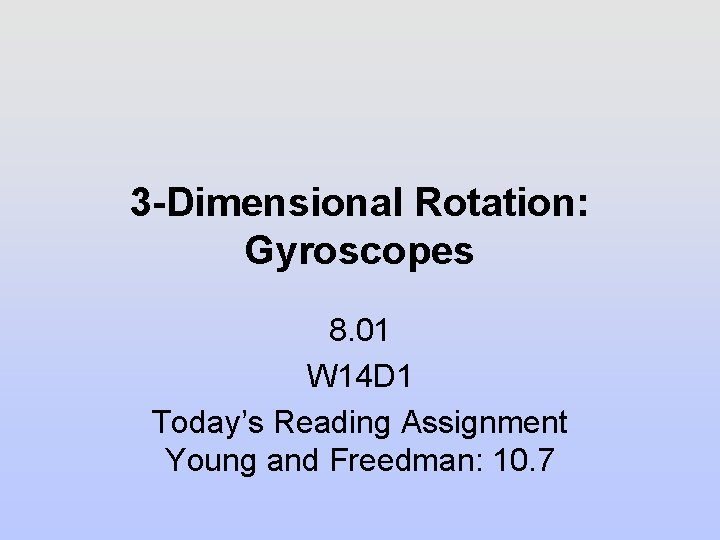3 -Dimensional Rotation: Gyroscopes 8. 01 W 14 D 1 Today’s Reading Assignment Young and Freedman: 10. 7Announcements Problem Set 11 Due Thursday Dec 8 9 pm Sunday Tutoring in 26 -152 from 1 -5 pm W 014 D 2 Reading Assignment Young and Freedman: 10. 7 2Demo: Gimbaled Gyroscope (B 140)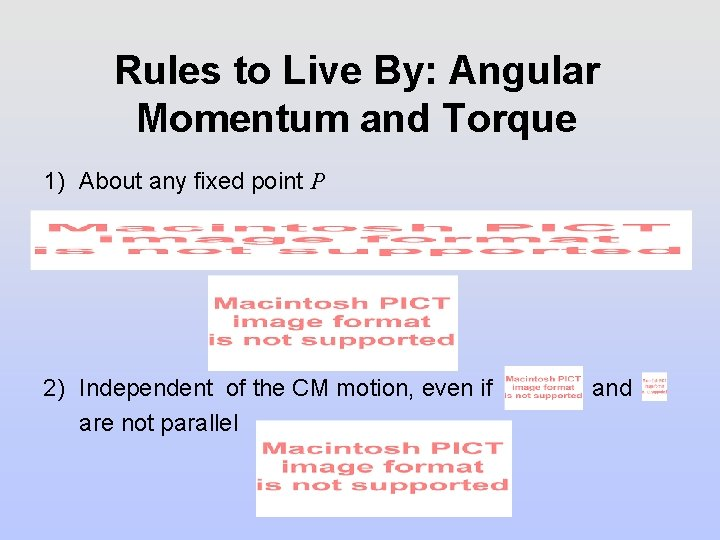Rules to Live By: Angular Momentum and Torque 1) About any fixed point P 2) Independent of the CM motion, even if are not parallel and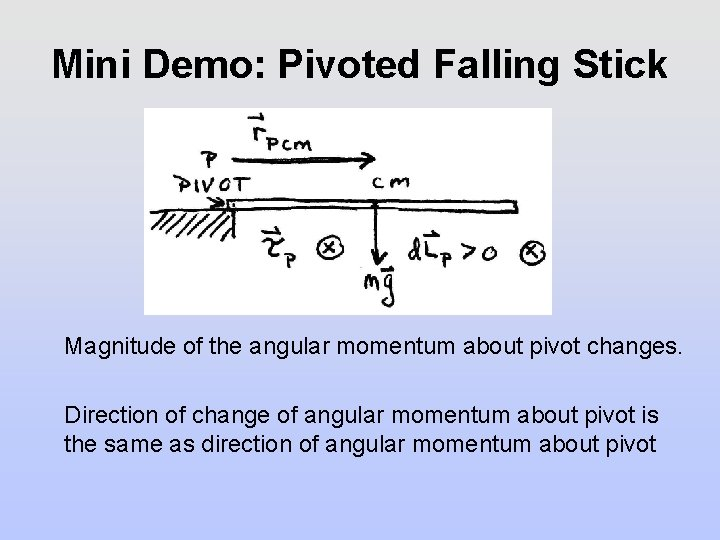Mini Demo: Pivoted Falling Stick Magnitude of the angular momentum about pivot changes. Direction of change of angular momentum about pivot is the same as direction of angular momentum about pivotDemo: Bicycle Wheel Two Cases Case 1: Magnitude of the angular momentum about pivot changes. Direction of change of angular momentum about pivot is the same as direction of angular momentum about pivot Case 2: Direction of angular momentum about pivot changes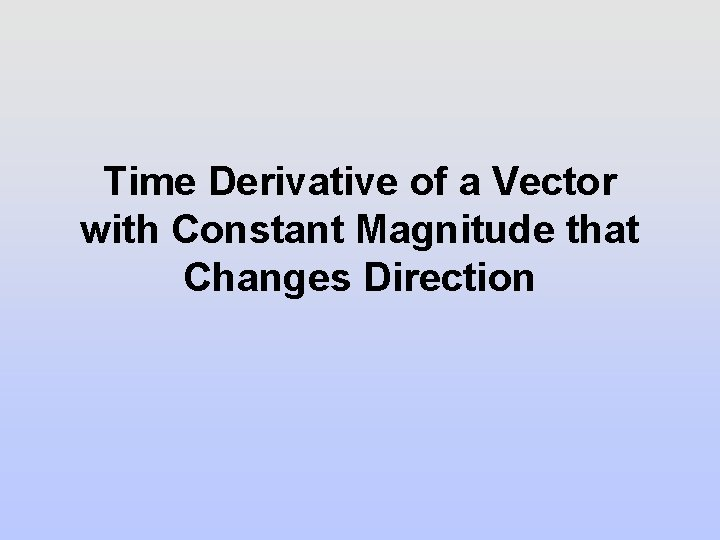Time Derivative of a Vector with Constant Magnitude that Changes Direction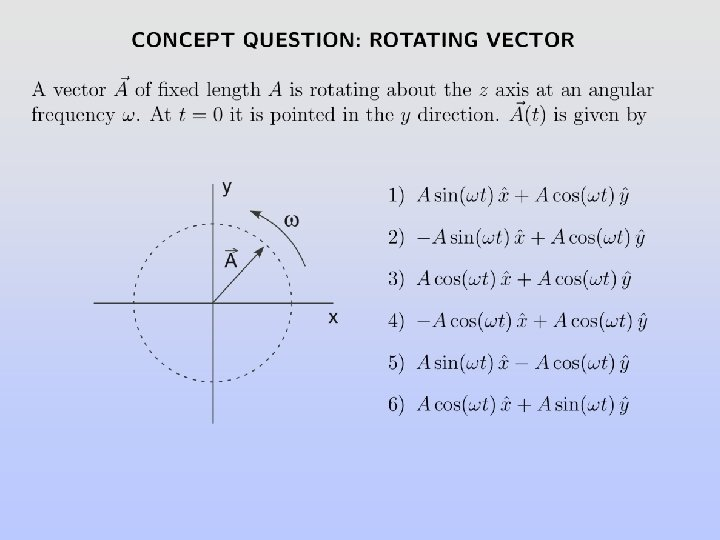Concept Question: Rotating Vector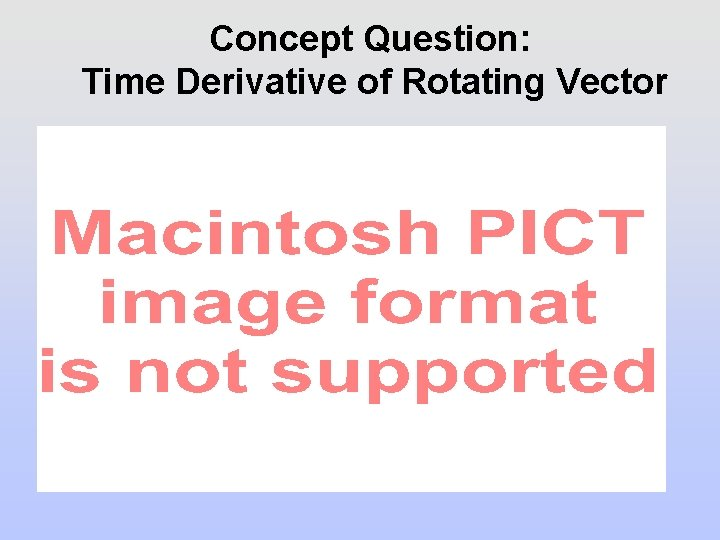Concept Question: Time Derivative of Rotating Vector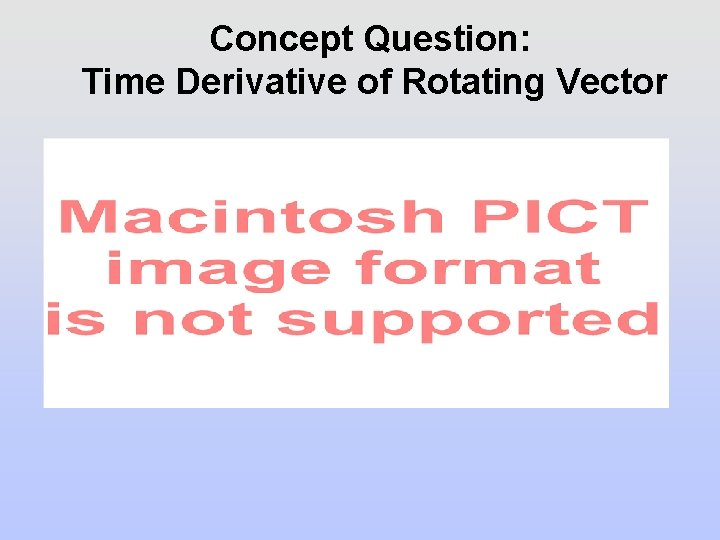Concept Question: Time Derivative of Rotating Vector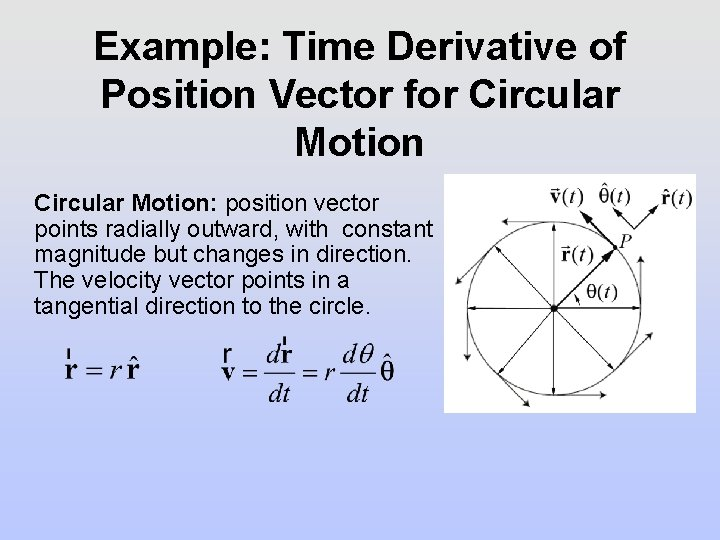Example: Time Derivative of Position Vector for Circular Motion: position vector points radially outward, with constant magnitude but changes in direction. The velocity vector points in a tangential direction to the circle.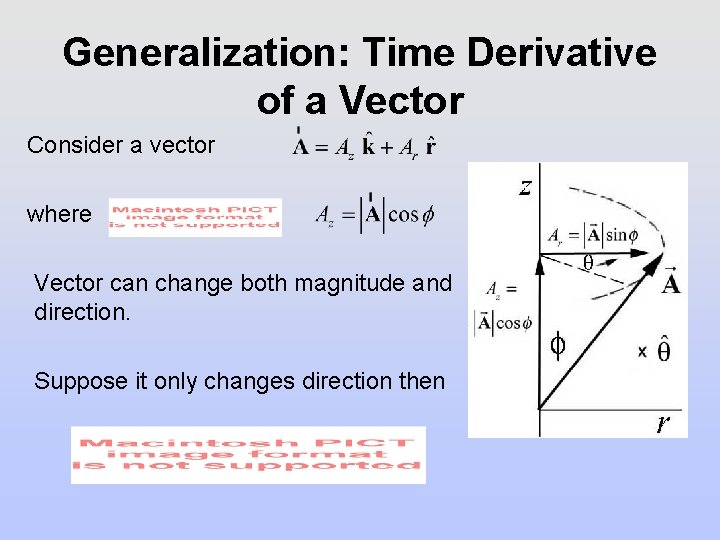Generalization: Time Derivative of a Vector Consider a vector where Vector can change both magnitude and direction. Suppose it only changes direction thenTorque and Time Derivative of Angular Momentum Torque about P is equal to the time derivative of the angular momentum about P If the magnitude of the angular momentum is constant then the torque can cause the direction of the perpendicular component of the angular momentum to change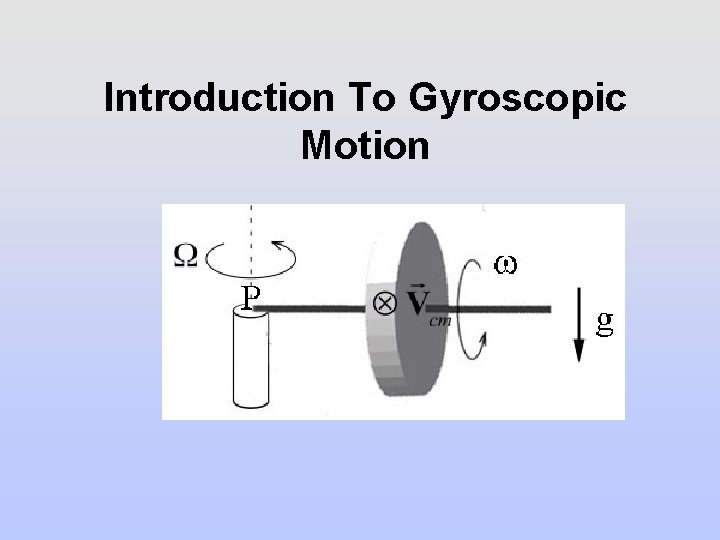Introduction To Gyroscopic MotionGyroscopic Approximation Flywheel is spinning with an angular velocity Precessional angular velocity Gyroscopic approximation: the angular velocity of precession is much less than the component of the spin angular velocity,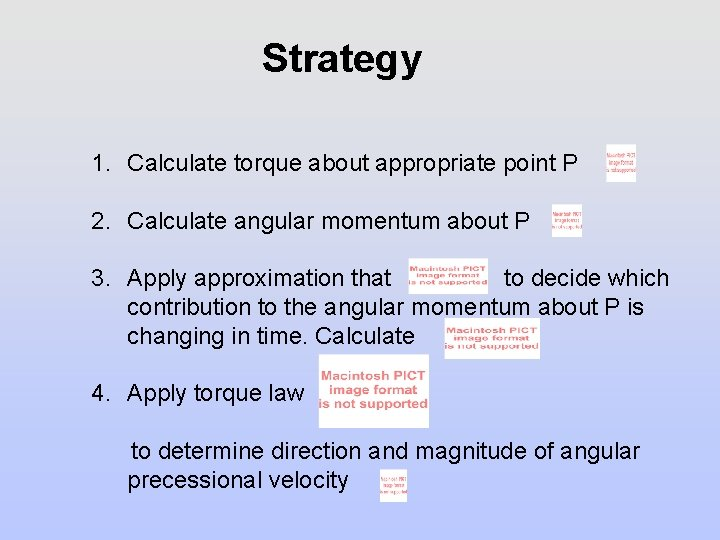Strategy 1. Calculate torque about appropriate point P 2. Calculate angular momentum about P 3. Apply approximation that to decide which contribution to the angular momentum about P is changing in time. Calculate 4. Apply torque law to determine direction and magnitude of angular precessional velocityTable Problem: Gyroscope: Forces and Torque Gravitational force acts at the center of the mass and points downward. Pivot force acts between the end of the axle and the pylon. What is the torque about the pivot point P due to gravitational forceIntroduction To Gyroscopic MotionGyroscopic Approximation Flywheel is spinning with an angular velocity Precessional angular velocity Gyroscopic approximation: the angular velocity of precession is much less than the component of the spin angular velocity,Strategy 1. Calculate torque about appropriate point P 2. Calculate angular momentum about P 3. Apply approximation that to decide which contribution to the angular momentum about P is changing in time. Calculate 4. Apply torque law to determine direction and magnitude of angular precessional velocity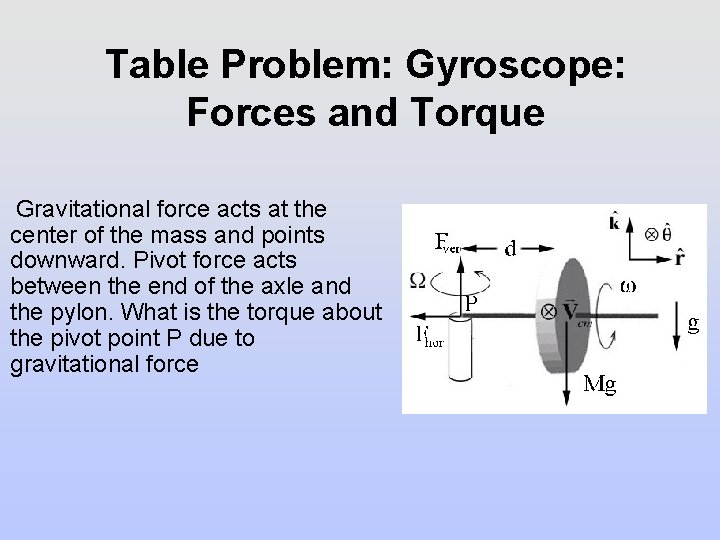Table Problem: Gyroscope: Forces and Torque Gravitational force acts at the center of the mass and points downward. Pivot force acts between the end of the axle and the pylon. What is the torque about the pivot point P due to gravitational force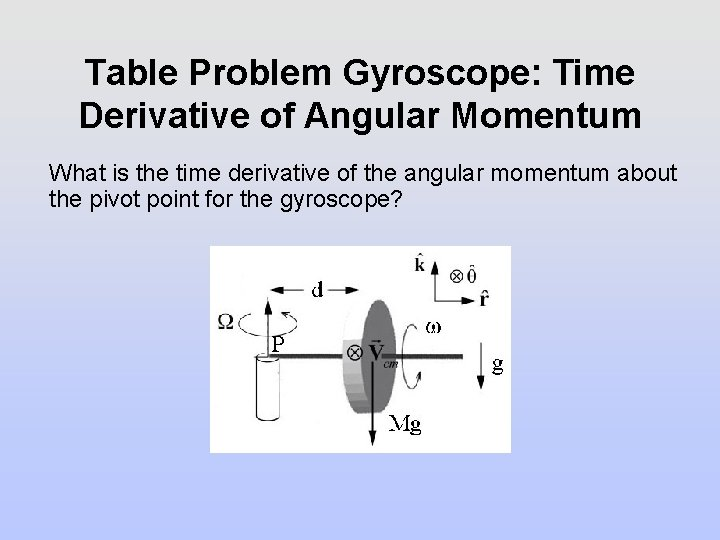Table Problem Gyroscope: Time Derivative of Angular Momentum What is the time derivative of the angular momentum about the pivot point for the gyroscope?Torque and Time Derivative of Angular Momentum Torque about P is equal to the time derivative of the angular momentum about P Therefore Precession angular speed isMore Detailed Analysis of Angular Momentum for Gyroscopic MotionAngular Momentum About Pivot Point The total angular momentum about the pivot point P of a horizontal gyroscope in steady state is the sum of the angular momentum due to the rotation about the center of mass and the angular momentum about the pivot point due to the center of mass motion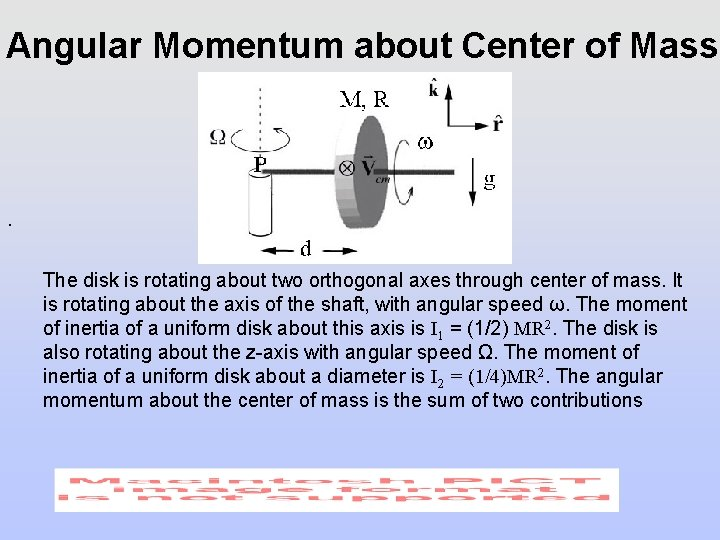Angular Momentum about Center of Mass . The disk is rotating about two orthogonal axes through center of mass. It is rotating about the axis of the shaft, with angular speed ω. The moment of inertia of a uniform disk about this axis is I 1 = (1/2) MR 2. The disk is also rotating about the z-axis with angular speed Ω. The moment of inertia of a uniform disk about a diameter is I 2 = (1/4)MR 2. The angular momentum about the center of mass is the sum of two contributions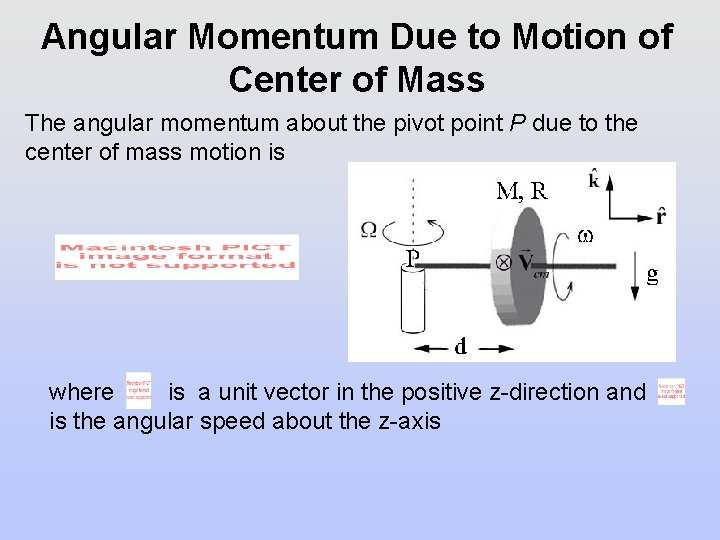Angular Momentum Due to Motion of Center of Mass The angular momentum about the pivot point P due to the center of mass motion is where is a unit vector in the positive z-direction and is the angular speed about the z-axis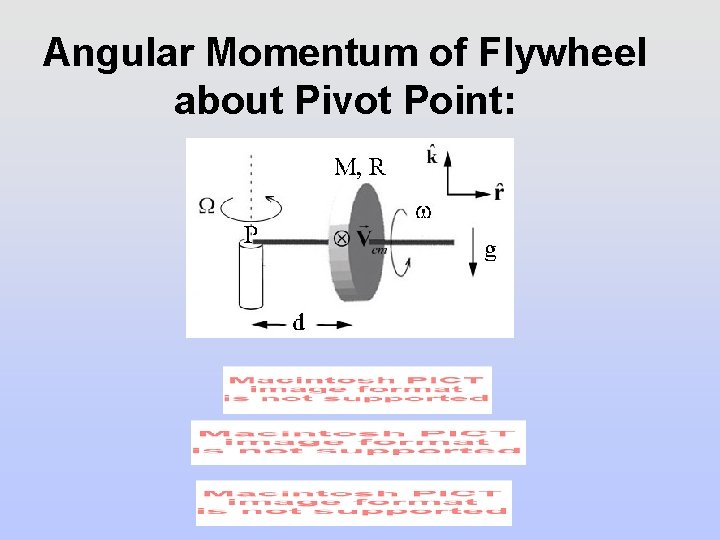Angular Momentum of Flywheel about Pivot Point: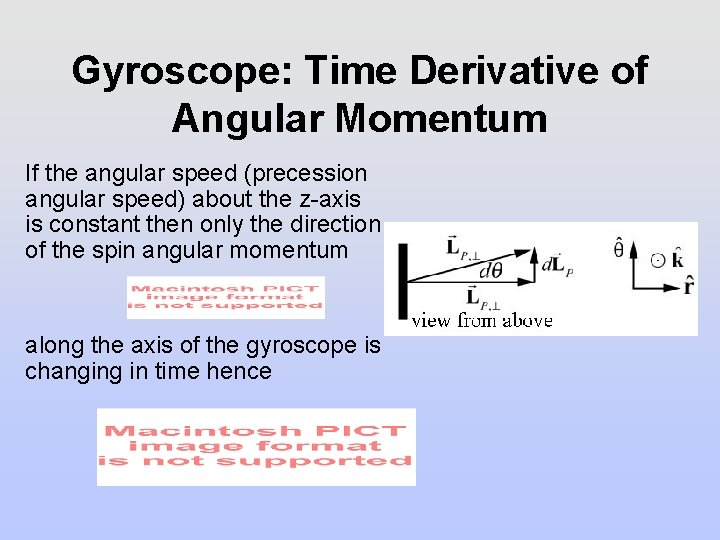Gyroscope: Time Derivative of Angular Momentum If the angular speed (precession angular speed) about the z-axis is constant then only the direction of the spin angular momentum along the axis of the gyroscope is changing in time henceConcept Question: Gyroscope For the simple gyroscope problem we just solved, if the mass of the disk is doubled how will the new precession rate Ω be related to the original rate Ω 0? 1) Ω = 4 Ω 0 2) Ω = 2 Ω 0 3) Ω = Ω 0 4) Ω = (1/2) Ω 0 5) Ω = (1/4) Ω 0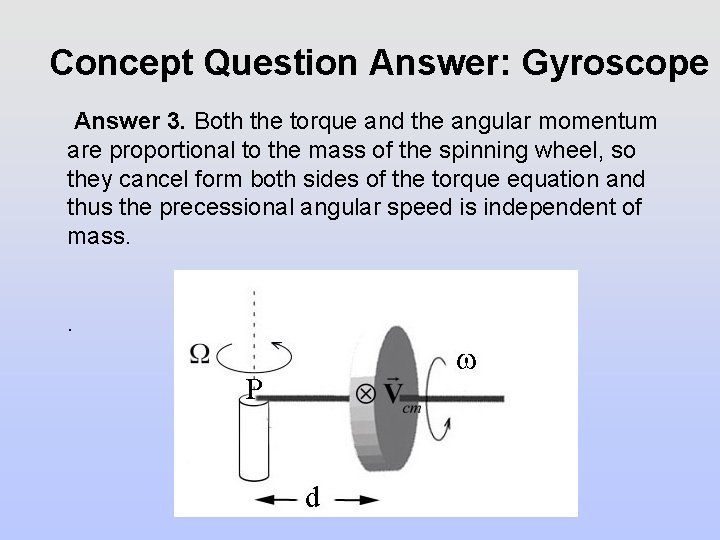Concept Question Answer: Gyroscope Answer 3. Both the torque and the angular momentum are proportional to the mass of the spinning wheel, so they cancel form both sides of the torque equation and thus the precessional angular speed is independent of mass. .Table Problem: Tilted Gyroscope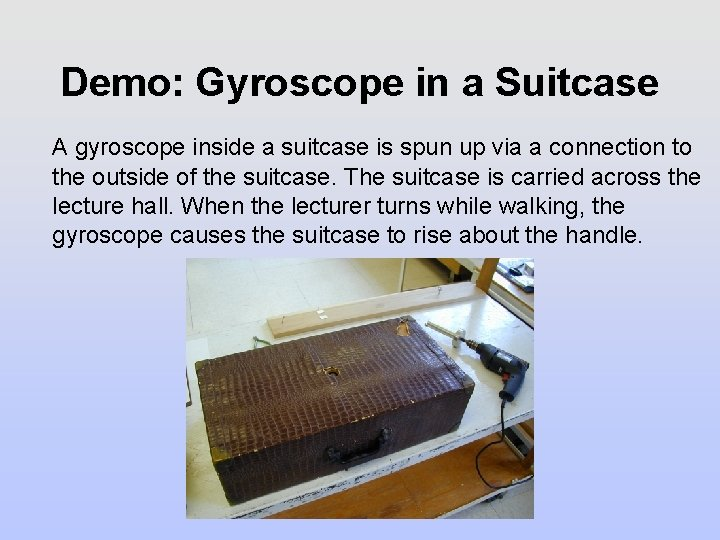Demo: Gyroscope in a Suitcase A gyroscope inside a suitcase is spun up via a connection to the outside of the suitcase. The suitcase is carried across the lecture hall. When the lecturer turns while walking, the gyroscope causes the suitcase to rise about the handle.Table Problem: Suspended GyroscopeTable Problem: Suspended Gyroscope